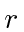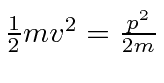## The Quantum Rotor

It is useful to simply investigate angular momentum with just one free rotation angle. This might be the quantum plane propeller. We will do a good job of this in 3 dimensions later.

Lets assume we have a massconstrained to move in a circle of radius. Assume the motion in the circle is free, so there is no potential. The kinetic energy isfor.

If we measure distance around the circle, thenand the one problem we have is that once I go completely around the circle, I am back to. Lets just go ahead and write our wavefunction.Remembering angular momentum, lets call the combination. Our wave is.

This must be single valued so we need to require thatThe angular momentum must be quantized in units of.

This will prove to be true for 3 dimensions too, however, the 3 components of angular momentum do not commute with each other, leading to all kinds of fun.

Jim Branson 2013-04-22